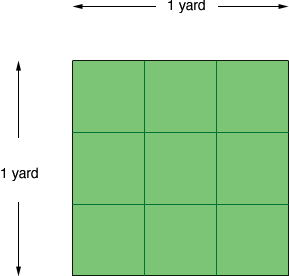Quandaries and Queries Hi, i'm another gardener that has forgotten how to calculate "how much topsoil in cubic yards i need to order to cover 7500sq. ft. 3 inches deep"? thanks Maggie Hi Maggie, A yard is three feet so a square that is one yard by one yard is 3 feet by three feet, and hence 33 = 9 square feet.Thus 7500 square feet is 7500/9 = 833.33 square yards. Three inches is 12/3 = 0.25 feet and hence 0.25/3 = 0.08333 yards. Thus you need 833.330.0833 = 69.4 cubic yards. Penny Go to Math Central